# Truncated tetraoctagonal tiling

Truncated tetraoctagonal tiling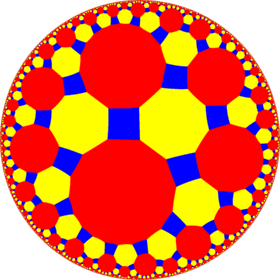Poincaré disk model of the hyperbolic plane
TypeHyperbolic uniform tiling
Vertex configuration4.8.16
Schläfli symboltr{8,4} orWythoff symbol2 8 4 |
Coxeter diagramor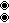Symmetry group[8,4], (*842)
DualOrder-4-8 kisrhombille tiling
PropertiesVertex-transitive

In geometry, the truncated tetraoctagonal tiling is a semiregular tiling of the hyperbolic plane. There are one square, one octagon, and one hexakaidecagon on each vertex. It has Schläfli symbol of tr{8,4}.

## Dual tiling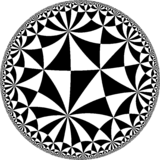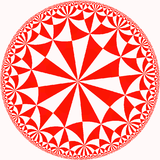The dual tiling is called an order-4-8 kisrhombille tiling, made as a complete bisection of the order-4 octagonal tiling, here with triangles are shown with alternating colors. This tiling represents the fundamental triangular domains of [8,4] (*842) symmetry.

### Symmetry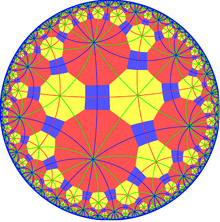Truncated tetraoctagonal tiling with *842,, mirror lines

There are 15 subgroups constructed from [8,4] by mirror removal and alternation. Mirrors can be removed if its branch orders are all even, and cuts neighboring branch orders in half. Removing two mirrors leaves a half-order gyration point where the removed mirrors met. In these images fundamental domains are alternately colored black and white, and mirrors exist on the boundaries between colors. The subgroup index-8 group, [1+,8,1+,4,1+] (4242) is the commutator subgroup of [8,4].

A larger subgroup is constructed as [8,4*], index 8, as [8,4+], (4*4) with gyration points removed, becomes (*4444) or (*44), and another [8*,4], index 16 as [8+,4], (8*2) with gyration points removed as (*22222222) or (*28). And their direct subgroups [8,4*]+, [8*,4]+, subgroup indices 16 and 32 respectively, can be given in orbifold notation as (4444) and (22222222).

From a Wythoff construction there are fourteen hyperbolic uniform tilings that can be based from the regular order-4 octagonal tiling.

Drawing the tiles colored as red on the original faces, yellow at the original vertices, and blue along the original edges, there are 7 forms with full [8,4] symmetry, and 7 with subsymmetry.Wikimedia Commons has media related to Uniform tiling 4-8-16.